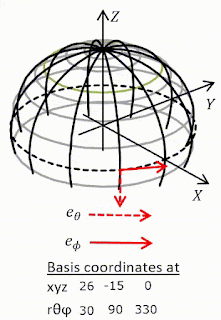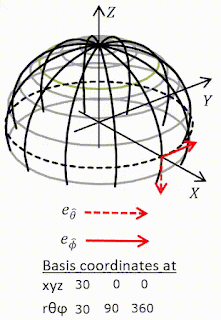## Tuesday 14 September 2021

### Question

Take the mathematician's view that tangent vectors and directional derivatives are the same thing, $u\equiv\partial_u$. Let $u,v$ be two vector fields and define their commutator in the manner familiar from quantum mechanics [not familiar to me!]$$\left[u,v\right]\equiv\left[\partial_u,\partial_v\right]\equiv\partial_u\partial_v-\partial_v\partial_u$$(a) Derive the following expression for $\left[u,v\right]$ valid in any coordinate basis$$\left[u,v\right]=\left(u^\beta v_{\ \ ,\beta}^\alpha-v^\beta u_{\ \ ,\beta}^\alpha\right)e_\alpha$$Thus despite that it looks like a second-order differential operator, $\left[u,v\right]$ is actually of first order - i.e. it is a tangent vector.
(b) For any basis $\left\{e_\alpha\right\}$ one defines the "commutation coefficients" $c_{\beta\gamma}^{\ \ \ \ \ \alpha}$ and $c_{\beta\gamma\alpha}$ by $$\left[e_\beta,e_\gamma\right]\equiv c_{\beta\gamma}^{\ \ \ \ \ \alpha}e_\alpha;\ \ c_{\beta\gamma\alpha}=g_{\alpha\mu}c_{\beta\gamma}^{\ \ \ \ \ \mu}$$Show that $c_{\beta\gamma}^{\ \ \ \ \ \alpha}=c_{\beta\gamma\alpha}=0$ got any coordinate basis.
(c) Calculate $c_{\hat{\beta}\hat{\gamma}}^{\ \ \ \ \ \hat{\alpha}}$ for the spherical noncoordinate basis of exercise 8.1.

This was straight forward. But I learned that Hermann Schwarz outshone Euler,  Lagrange, Cauchy who were all very clever. It was Schwarz who proved that partial derivatives commute. The others didn't get it quite right apparently. So I repeated his proof and now I know when partial derivatives commute and when they don't. Hurrah.
On this exercise: 8.2 Exercise Commutators.pdf (3 pages)
On Schwarz's theorem: 8.2 Schwarz's theorem.pdf (4 pages)

## Thursday 9 September 2021

### MTW Ex 8.1 Practice with tensor algebra

#### Question

Let $t,x,y,z$ be Lorentz coordinates in flat spacetime, and let $$r=\left(x^2+y^2+z^2\right)^\frac{1}{2}\ ,\ \theta=\arccos{\left(\frac{z}{r}\right)}\ ,\ \phi=\arctan{\left(\frac{y}{x}\right)}$$be the corresponding spherical coordinates. Then$$e_0=\frac{\partial\mathcal{P}}{\partial t}\ ,\ e_r=\frac{\partial\mathcal{P}}{\partial r}\ ,\ e_\theta=\frac{\partial\mathcal{P}}{\partial\theta}\ ,\ e_\phi=\frac{\partial\mathcal{P}}{\partial\phi}$$is a coordinate basis, and $$e_{\hat{0}}=\frac{\partial\mathcal{P}}{\partial t}\ ,\ e_{\hat{r}}=\frac{\partial\mathcal{P}}{\partial r}\ ,\ e_{\hat{\theta}}=\frac{1}{r}\frac{\partial\mathcal{P}}{\partial\theta}\ ,\ e_{\hat{\phi}}=\frac{1}{r\sin{\theta}}\frac{\partial\mathcal{P}}{\partial\phi}$$is a non-coordinate basis.
(a) Draw a picture of $e_\theta,e_\phi,e_{\hat{\theta}},e_{\hat{\phi}}$ at several different points on a sphere of constant $t,r$.
(b) What are the one-form bases dual to these tangent-vector bases?
(c) What is the transformation matrix linking the original Lorentz frame to the spherical coordinate frame $\left\{e_a\right\}$?
(d) Use this transformation matrix to calculate the metric components $g_{\alpha\beta}$ in the spherical coordinate basis and invert the result to get $g^{\alpha\beta}$.
(e) Show that the non-coordinate basis $\left\{e_{\hat{a}}\right\}$ is orthonormal everywhere; i.e. that $g_{\hat{\alpha}\hat{\beta}}=\eta_{\alpha\beta}$; i.e. that $$g=-\omega^{\hat{0}}\otimes\omega^{\hat{0}}+\omega^{\hat{r}}\otimes\omega^{\hat{r}}+\omega^{\hat{\theta}}\otimes\omega^{\hat{\theta}}+\omega^{\hat{\phi}}\otimes\omega^{\hat{\phi}}$$(f) Write the gradient of a function $f$ in terms of the spherical coordinate and noncoordinate bases.
(g) What are the components of the Levi-Civita tensor in the spherical coordinate and noncoordinate bases?

This exercise seems mainly to be concerned with putting the rules in Box 8.4 into practice. We meet a noncoordinate (anholonomic) basis. One of the features of these is that coordinates cannot be used to describe positions but you can use components to describe other tensors. So$$p^{\hat{\mu}}=\left(a,b,c,d\right)\equiv ae^{\hat{0}}+be^{\hat{r}}+ce^{\hat{\theta}}+de^{\hat{\phi}}$$is at best meaningless but$$g_{\hat{\alpha}\hat{\beta}}=\left(\begin{matrix}a&0&0&0\\0&b&0&0\\0&0&c&0\\0&0&0&d\\\end{matrix}\right)$$is valid and is the answer to (d) when $a=-1,b=c=d=1$ .
I found an excellent new way of  creating animated gifs. Which I used for the answer to (a) below. The diagrams for $e_{\hat{\theta}},e_{\hat{\phi}}$ are a good indication of (e).
 Coordinate bases going round equatorCoordinate bases going over poleNon-coordinate bases going round equatorNon-coordinate bases going round equator